# 3.1 Complex numbers  (Page 4/8)

 Page 4 / 8

## Dividing complex numbers

Divide $\text{\hspace{0.17em}}\left(2+5i\right)\text{\hspace{0.17em}}$ by $\text{\hspace{0.17em}}\left(4-i\right).$

We begin by writing the problem as a fraction.

$\frac{\left(2+5i\right)}{\left(4-i\right)}$

Then we multiply the numerator and denominator by the complex conjugate of the denominator.

$\frac{\left(2+5i\right)}{\left(4-i\right)}\cdot \frac{\left(4+i\right)}{\left(4+i\right)}$

To multiply two complex numbers, we expand the product as we would with polynomials (the process commonly called FOIL).

Note that this expresses the quotient in standard form.

## Substituting a complex number into a polynomial function

Let $\text{\hspace{0.17em}}f\left(x\right)={x}^{2}-5x+2.\text{\hspace{0.17em}}$ Evaluate $\text{\hspace{0.17em}}f\left(3+i\right).$

Substitute $\text{\hspace{0.17em}}x=3+i\text{\hspace{0.17em}}$ into the function $\text{\hspace{0.17em}}f\left(x\right)={x}^{2}-5x+2\text{\hspace{0.17em}}$ and simplify.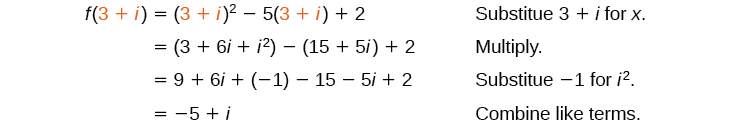Let $\text{\hspace{0.17em}}f\left(x\right)=2{x}^{2}-3x.\text{\hspace{0.17em}}$ Evaluate $\text{\hspace{0.17em}}f\left(8-i\right).$

$102-29i$

## Substituting an imaginary number in a rational function

Let $\text{\hspace{0.17em}}f\left(x\right)=\frac{2+x}{x+3}.\text{\hspace{0.17em}}$ Evaluate $\text{\hspace{0.17em}}f\left(10i\right).$

Substitute $\text{\hspace{0.17em}}x=10i\text{\hspace{0.17em}}$ and simplify.

Let $\text{\hspace{0.17em}}f\left(x\right)=\frac{x+1}{x-4}.\text{\hspace{0.17em}}$ Evaluate $\text{\hspace{0.17em}}f\left(-i\right).$

$-\frac{3}{17}+\frac{5i}{17}$

## Simplifying powers of i

The powers of $\text{\hspace{0.17em}}i\text{\hspace{0.17em}}$ are cyclic. Let’s look at what happens when we raise $\text{\hspace{0.17em}}i\text{\hspace{0.17em}}$ to increasing powers.

$\begin{array}{l}{i}^{1}=i\\ {i}^{2}=-1\\ {i}^{3}={i}^{2}\cdot i=-1\cdot i=-i\\ {i}^{4}={i}^{3}\cdot i=-i\cdot i=-{i}^{2}=-\left(-1\right)=1\\ {i}^{5}={i}^{4}\cdot i=1\cdot i=i\end{array}$

We can see that when we get to the fifth power of $\text{\hspace{0.17em}}i,\text{\hspace{0.17em}}$ it is equal to the first power. As we continue to multiply $\text{\hspace{0.17em}}i\text{\hspace{0.17em}}$ by itself for increasing powers, we will see a cycle of 4. Let’s examine the next 4 powers of $\text{\hspace{0.17em}}i.$

$\begin{array}{l}{i}^{6}={i}^{5}\cdot i=i\cdot i={i}^{2}=-1\\ {i}^{7}={i}^{6}\cdot i={i}^{2}\cdot i={i}^{3}=-i\\ {i}^{8}={i}^{7}\cdot i={i}^{3}\cdot i={i}^{4}=1\\ {i}^{9}={i}^{8}\cdot i={i}^{4}\cdot i={i}^{5}=i\end{array}$

## Simplifying powers of $\text{\hspace{0.17em}}i$

Evaluate $\text{\hspace{0.17em}}{i}^{35}.$

Since $\text{\hspace{0.17em}}{i}^{4}=1,\text{\hspace{0.17em}}$ we can simplify the problem by factoring out as many factors of $\text{\hspace{0.17em}}{i}^{4}\text{\hspace{0.17em}}$ as possible. To do so, first determine how many times 4 goes into 35: $\text{\hspace{0.17em}}35=4\cdot 8+3.$

${i}^{35}={i}^{4\cdot 8+3}={i}^{4\cdot 8}\cdot {i}^{3}={\left({i}^{4}\right)}^{8}\cdot {i}^{3}={1}^{8}\cdot {i}^{3}={i}^{3}=-i$

Can we write $\text{\hspace{0.17em}}{i}^{35}\text{\hspace{0.17em}}$ in other helpful ways?

As we saw in [link] , we reduced $\text{\hspace{0.17em}}{i}^{35}\text{\hspace{0.17em}}$ to $\text{\hspace{0.17em}}{i}^{3}\text{\hspace{0.17em}}$ by dividing the exponent by 4 and using the remainder to find the simplified form. But perhaps another factorization of $\text{\hspace{0.17em}}{i}^{35}\text{\hspace{0.17em}}$ may be more useful. [link] shows some other possible factorizations.

 Factorization of $\text{\hspace{0.17em}}{i}^{35}$ ${i}^{34}\cdot i$ ${i}^{33}\cdot {i}^{2}$ ${i}^{31}\cdot {i}^{4}$ ${i}^{19}\cdot {i}^{16}$ Reduced form ${\left({i}^{2}\right)}^{17}\cdot i$ ${i}^{33}\cdot \left(-1\right)$ ${i}^{31}\cdot 1$ ${i}^{19}\cdot {\left({i}^{4}\right)}^{4}$ Simplified form ${\left(-1\right)}^{17}\cdot i$ $-{i}^{33}$ ${i}^{31}$ ${i}^{19}$

Each of these will eventually result in the answer we obtained above but may require several more steps than our earlier method.

Access these online resources for additional instruction and practice with complex numbers.

## Key concepts

• The square root of any negative number can be written as a multiple of $\text{\hspace{0.17em}}i.\text{\hspace{0.17em}}$ See [link] .
• To plot a complex number, we use two number lines, crossed to form the complex plane. The horizontal axis is the real axis, and the vertical axis is the imaginary axis. See [link] .
• Complex numbers can be added and subtracted by combining the real parts and combining the imaginary parts. See [link] .
• Complex numbers can be multiplied and divided.
• To multiply complex numbers, distribute just as with polynomials. See [link] , [link] , and [link] .
• To divide complex numbers, multiply both the numerator and denominator by the complex conjugate of the denominator to eliminate the complex number from the denominator. See [link] , [link] , and [link] .
• The powers of $\text{\hspace{0.17em}}i\text{\hspace{0.17em}}$ are cyclic, repeating every fourth one. See [link] .

## Verbal

Explain how to add complex numbers.

Add the real parts together and the imaginary parts together.

What is the basic principle in multiplication of complex numbers?

Give an example to show the product of two imaginary numbers is not always imaginary.

$i\text{\hspace{0.17em}}$ times $\text{\hspace{0.17em}}i\text{\hspace{0.17em}}$ equals –1, which is not imaginary. (answers vary)

What is a characteristic of the plot of a real number in the complex plane?

## Algebraic

For the following exercises, evaluate the algebraic expressions.

evaluate $\text{\hspace{0.17em}}f\left(2i\right).$

$-8+2i$

evaluate $\text{\hspace{0.17em}}f\left(i\right).$

evaluate $\text{\hspace{0.17em}}f\left(2+i\right).$

$14+7i$

evaluate $\text{\hspace{0.17em}}f\left(2-3i\right).$

evaluate $\text{\hspace{0.17em}}f\left(5i\right).$

$-\frac{23}{29}+\frac{15}{29}i$

evaluate $\text{\hspace{0.17em}}f\left(4i\right).$

## Graphical

For the following exercises, determine the number of real and nonreal solutions for each quadratic function shown.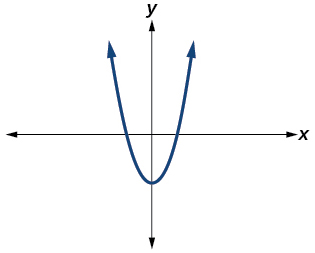2 real and 0 nonreal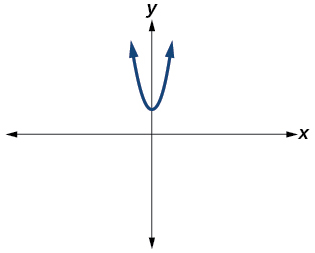For the following exercises, plot the complex numbers on the complex plane.

$1-2i$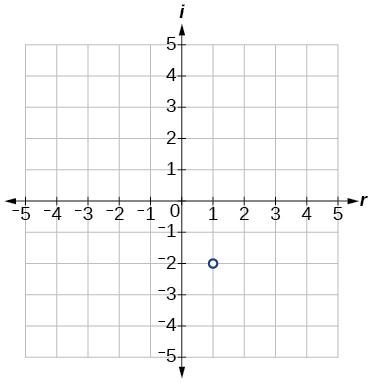$-2+3i$

$i$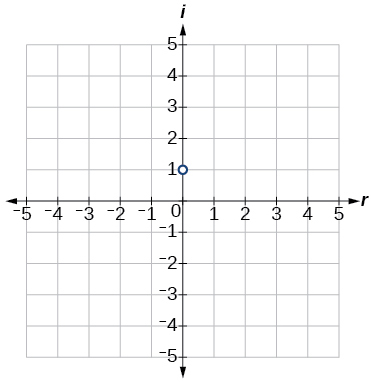$-3-4i$

## Numeric

For the following exercises, perform the indicated operation and express the result as a simplified complex number.

$\left(3+2i\right)+\left(5-3i\right)$

$8-i$

$\left(-2-4i\right)+\left(1+6i\right)$

$\left(-5+3i\right)-\left(6-i\right)$

$-11+4i$

$\left(2-3i\right)-\left(3+2i\right)$

$\left(-4+4i\right)-\left(-6+9i\right)$

$2-5i$

$\left(2+3i\right)\left(4i\right)$

$\left(5-2i\right)\left(3i\right)$

$6+15i$

$\left(6-2i\right)\left(5\right)$

$\left(-2+4i\right)\left(8\right)$

$-16+32i$

$\left(2+3i\right)\left(4-i\right)$

$\left(-1+2i\right)\left(-2+3i\right)$

$-4-7i$

$\left(4-2i\right)\left(4+2i\right)$

$\left(3+4i\right)\left(3-4i\right)$

25

$\frac{3+4i}{2}$

$\frac{6-2i}{3}$

$2-\frac{2}{3}i$

$\frac{-5+3i}{2i}$

$\frac{6+4i}{i}$

$4-6i$

$\frac{2-3i}{4+3i}$

$\frac{3+4i}{2-i}$

$\frac{2}{5}+\frac{11}{5}i$

$\frac{2+3i}{2-3i}$

$\sqrt{-9}+3\sqrt{-16}$

$15i$

$-\sqrt{-4}-4\sqrt{-25}$

$\frac{2+\sqrt{-12}}{2}$

$1+i\sqrt{3}$

$\frac{4+\sqrt{-20}}{2}$

${i}^{8}$

$1$

${i}^{15}$

${i}^{22}$

$-1$

## Technology

For the following exercises, use a calculator to help answer the questions.

Evaluate $\text{\hspace{0.17em}}{\left(1+i\right)}^{k}\text{\hspace{0.17em}}$ for Predict the value if $\text{\hspace{0.17em}}k=16.$

Evaluate $\text{\hspace{0.17em}}{\left(1-i\right)}^{k}\text{\hspace{0.17em}}$ for Predict the value if $\text{\hspace{0.17em}}k=14.$

128i

Evaluate $\text{\hspace{0.17em}}\left(1+i{\right)}^{k}-\left(1-i{\right)}^{k}$ for . Predict the value for $\text{\hspace{0.17em}}k=16.$

Show that a solution of $\text{\hspace{0.17em}}{x}^{6}+1=0\text{\hspace{0.17em}}$ is $\text{\hspace{0.17em}}\frac{\sqrt{3}}{2}+\frac{1}{2}i.$

${\left(\frac{\sqrt{3}}{2}+\frac{1}{2}i\right)}^{6}=-1$

Show that a solution of $\text{\hspace{0.17em}}{x}^{8}-1=0\text{\hspace{0.17em}}$ is $\text{\hspace{0.17em}}\frac{\sqrt{2}}{2}+\frac{\sqrt{2}}{2}i.$

## Extensions

For the following exercises, evaluate the expressions, writing the result as a simplified complex number.

$\frac{1}{i}+\frac{4}{{i}^{3}}$

$3i$

$\frac{1}{{i}^{11}}-\frac{1}{{i}^{21}}$

${i}^{7}\left(1+{i}^{2}\right)$

0

${i}^{-3}+5{i}^{7}$

$\frac{\left(2+i\right)\left(4-2i\right)}{\left(1+i\right)}$

5 – 5i

$\frac{\left(1+3i\right)\left(2-4i\right)}{\left(1+2i\right)}$

$\frac{{\left(3+i\right)}^{2}}{{\left(1+2i\right)}^{2}}$

$-2i$

$\frac{3+2i}{2+i}+\left(4+3i\right)$

$\frac{4+i}{i}+\frac{3-4i}{1-i}$

$\frac{9}{2}-\frac{9}{2}i$

$\frac{3+2i}{1+2i}-\frac{2-3i}{3+i}$

#### Questions & Answers

x=-b+_Гb2-(4ac) ______________ 2a
I've run into this: x = r*cos(angle1 + angle2) Which expands to: x = r(cos(angle1)*cos(angle2) - sin(angle1)*sin(angle2)) The r value confuses me here, because distributing it makes: (r*cos(angle2))(cos(angle1) - (r*sin(angle2))(sin(angle1)) How does this make sense? Why does the r distribute once
so good
abdikarin
this is an identity when 2 adding two angles within a cosine. it's called the cosine sum formula. there is also a different formula when cosine has an angle minus another angle it's called the sum and difference formulas and they are under any list of trig identities
How can you tell what type of parent function a graph is ?
generally by how the graph looks and understanding what the base parent functions look like and perform on a graph
William
if you have a graphed line, you can have an idea by how the directions of the line turns, i.e. negative, positive, zero
William
y=x will obviously be a straight line with a zero slope
William
y=x^2 will have a parabolic line opening to positive infinity on both sides of the y axis vice versa with y=-x^2 you'll have both ends of the parabolic line pointing downward heading to negative infinity on both sides of the y axis
William
y=x will be a straight line, but it will have a slope of one. Remember, if y=1 then x=1, so for every unit you rise you move over positively one unit. To get a straight line with a slope of 0, set y=1 or any integer.
Aaron
yes, correction on my end, I meant slope of 1 instead of slope of 0
William
what is f(x)=
I don't understand
Joe
Typically a function 'f' will take 'x' as input, and produce 'y' as output. As 'f(x)=y'. According to Google, "The range of a function is the complete set of all possible resulting values of the dependent variable (y, usually), after we have substituted the domain."
Thomas
Sorry, I don't know where the "Â"s came from. They shouldn't be there. Just ignore them. :-)
Thomas
Darius
Thanks.
Thomas
Â
Thomas
It is the Â that should not be there. It doesn't seem to show if encloses in quotation marks. "Â" or 'Â' ... Â
Thomas
Now it shows, go figure?
Thomas
what is this?
i do not understand anything
unknown
lol...it gets better
Darius
I've been struggling so much through all of this. my final is in four weeks 😭
Tiffany
this book is an excellent resource! have you guys ever looked at the online tutoring? there's one that is called "That Tutor Guy" and he goes over a lot of the concepts
Darius
thank you I have heard of him. I should check him out.
Tiffany
is there any question in particular?
Joe
I have always struggled with math. I get lost really easy, if you have any advice for that, it would help tremendously.
Tiffany
Sure, are you in high school or college?
Darius
Hi, apologies for the delayed response. I'm in college.
Tiffany
how to solve polynomial using a calculator
So a horizontal compression by factor of 1/2 is the same as a horizontal stretch by a factor of 2, right?
The center is at (3,4) a focus is at (3,-1), and the lenght of the major axis is 26
The center is at (3,4) a focus is at (3,-1) and the lenght of the major axis is 26 what will be the answer?
Rima
I done know
Joe
What kind of answer is that😑?
Rima
I had just woken up when i got this message
Joe
Can you please help me. Tomorrow is the deadline of my assignment then I don't know how to solve that
Rima
i have a question.
Abdul
how do you find the real and complex roots of a polynomial?
Abdul
@abdul with delta maybe which is b(square)-4ac=result then the 1st root -b-radical delta over 2a and the 2nd root -b+radical delta over 2a. I am not sure if this was your question but check it up
Nare
This is the actual question: Find all roots(real and complex) of the polynomial f(x)=6x^3 + x^2 - 4x + 1
Abdul
@Nare please let me know if you can solve it.
Abdul
I have a question
juweeriya
hello guys I'm new here? will you happy with me
mustapha
The average annual population increase of a pack of wolves is 25.
how do you find the period of a sine graph
Period =2π if there is a coefficient (b), just divide the coefficient by 2π to get the new period
Am
if not then how would I find it from a graph
Imani
by looking at the graph, find the distance between two consecutive maximum points (the highest points of the wave). so if the top of one wave is at point A (1,2) and the next top of the wave is at point B (6,2), then the period is 5, the difference of the x-coordinates.
Am
you could also do it with two consecutive minimum points or x-intercepts
Am
I will try that thank u
Imani
Case of Equilateral Hyperbola
ok
Zander
ok
Shella
f(x)=4x+2, find f(3)
Benetta
f(3)=4(3)+2 f(3)=14
lamoussa
14
Vedant
pre calc teacher: "Plug in Plug in...smell's good" f(x)=14
Devante
8x=40
Chris
Explain why log a x is not defined for a < 0
the sum of any two linear polynomial is what
divide simplify each answer 3/2÷5/4
divide simplify each answer 25/3÷5/12
Momo
how can are find the domain and range of a relations
the range is twice of the natural number which is the domain
MorolakeByByByByBy David Martin